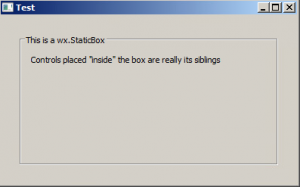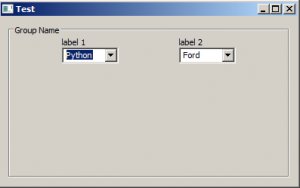# An Intro to StaticBox and StaticBoxSizers

There are many widgets that are included with the wxPython GUI toolkit. One of them is a fairly handy widget called wx.StaticBox. This widget accepts a string and then will draw a box with the string in the upper left-hand corned of the box. However this only works when you use it in conjunction with wx.StaticBoxSizer.

Here is an example of what one might look like:Now let’s go ahead and write the code you would use to create the example above:

```import wx

class MyPanel(wx.Panel):

def __init__(self, parent):
super().__init__(parent)
box = wx.StaticBox(self, -1, "This is a wx.StaticBox")
bsizer = wx.StaticBoxSizer(box, wx.VERTICAL)

t = wx.StaticText(self, -1, "Controls placed \"inside\" the box are really its siblings")

border = wx.BoxSizer()
self.SetSizer(border)

class MyFrame(wx.Frame):

def __init__(self):
super().__init__(None, title='Test')
panel = MyPanel(self)
self.Show()

if __name__ == '__main__':
app = wx.App(False)
frame = MyFrame()
app.MainLoop()```

This code is based on the wx.StaticBox demo code from wxPython’s demo application. Basically you create the wx.StaticBox, add it to an instance of wx.StaticBoxSizer and then add that to a wx.BoxSizer and you’re done.

But what if you wanted a more complicated layout within the box?

Let’s take a look at that use-case next!

### Nesting Sizers in wx.StaticBoxSizer

More often then not, you will want more than a single widget inside of your box widget. When that happens, you will need to use sizers inside of your wx.StaticBoxSizer.

Here is an example:Let’s go ahead and take a look at the code for this example:

```import wx

class MyPanel(wx.Panel):

def __init__(self, parent):
super().__init__(parent)

box = wx.StaticBox(self, -1, "Group Name")
bsizer = wx.StaticBoxSizer(box, wx.VERTICAL)

hsizer = wx.BoxSizer()

vsizer = wx.BoxSizer(wx.VERTICAL)
lbl = wx.StaticText(self, label='label 1')
cbo = wx.ComboBox(self, value='Python', choices=['Python', 'Ruby'],
size=(75, -1))

vsizer = wx.BoxSizer(wx.VERTICAL)
lbl = wx.StaticText(self, label='label 2')
cbo = wx.ComboBox(self, value='Ford', choices=['Ford', 'Chevrolet'],
size=(75, -1))

main_sizer = wx.BoxSizer()
main_sizer.Add(bsizer, 1, wx.EXPAND | wx.ALL, 10)
self.SetSizer(main_sizer)

class MyFrame(wx.Frame):

def __init__(self):
super().__init__(None, title='Test')
panel = MyPanel(self)
self.Show()

if __name__ == '__main__':
app = wx.App(False)
frame = MyFrame()
app.MainLoop()```

In this case, you need to create two vertically oriented wx.BoxSizers to hold the four widgets in two columns. You add those sizers to a horizontally oriented wx.BoxSizer as well. If you wanted to simplify this a bit, you could use a wx.GridSizer instead of these BoxSizers.

Regardless of the approach, you end up with a nicely laid out application.

### Wrapping Up

Using the wx.StaticBox widget is pretty straight-forward overall. I think it could be simpler if the widget and the sizer were combined into one class though. Anyway, if you’d like to learn more about this widget, you should see the documentation. Have fun and happy coding!